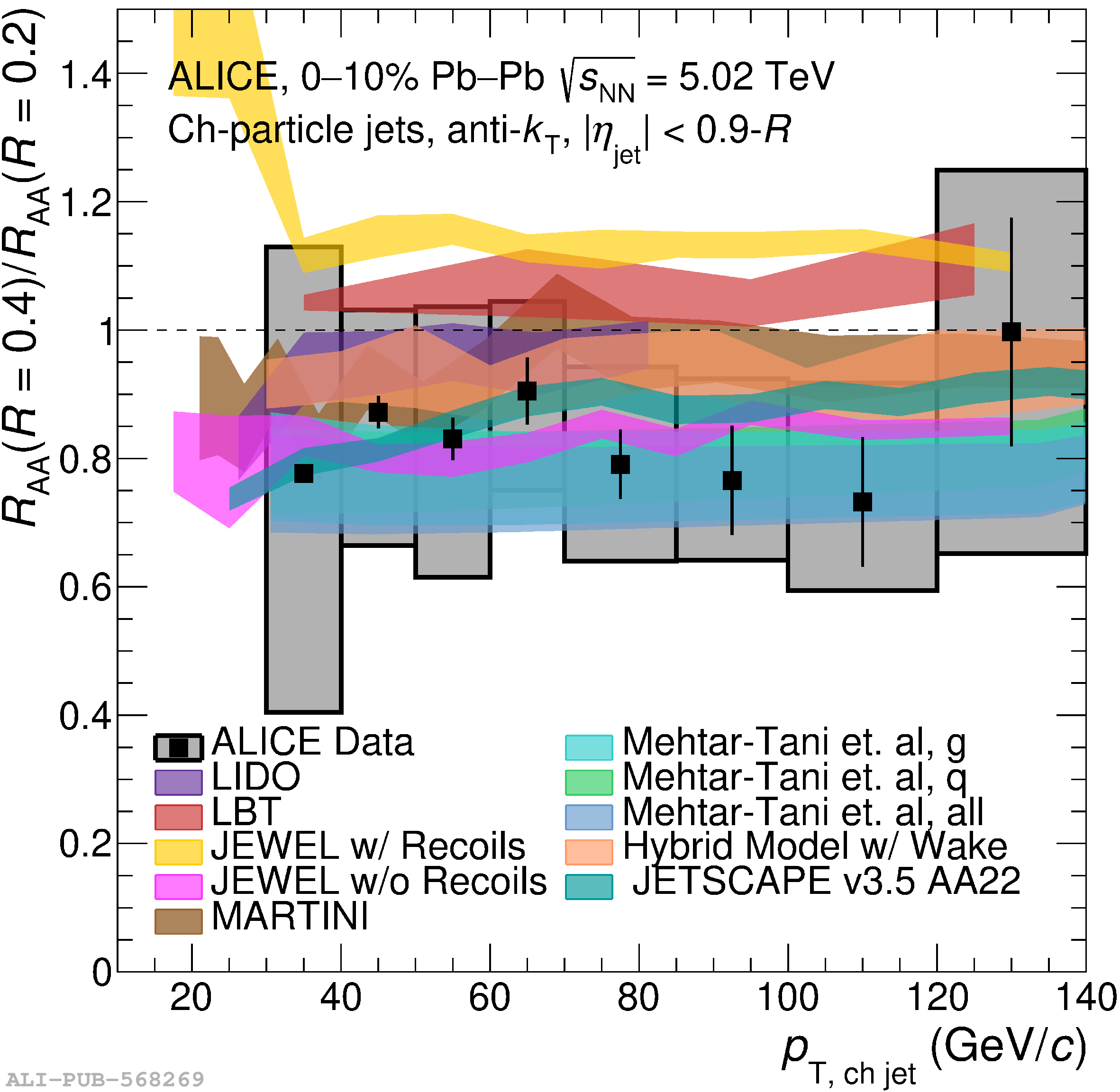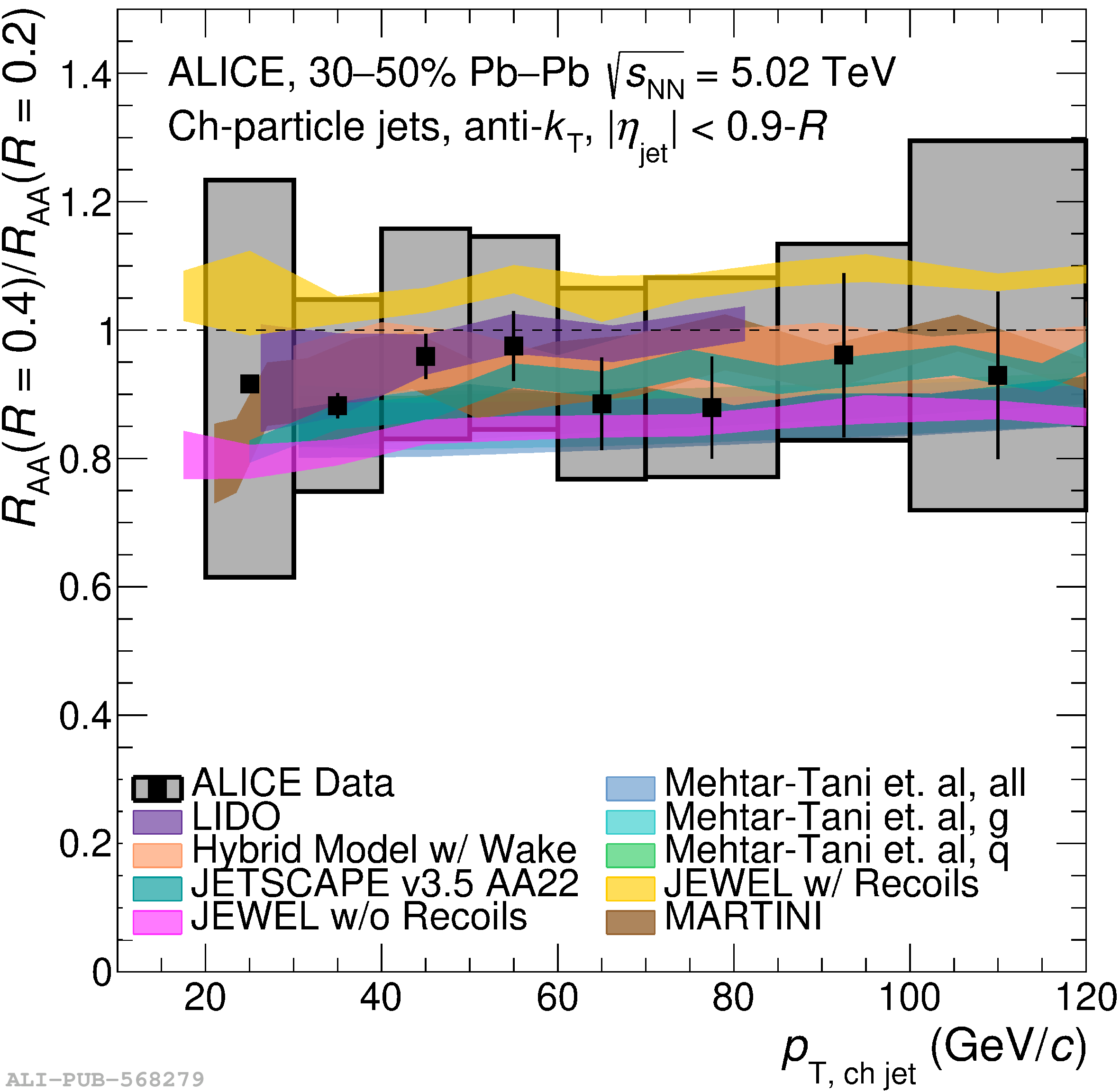# Figure 8

 Double ratio of jet nuclear modification factors using $R_\mathrm{AA}^{R=0.2}$ as denominator and using $R = 0.4$ (left) and $R = 0.6$ (right) as the numerator compared to model predictions for central (top row) and semi-central (bottom row) collisions. Note that a comparison to JEWEL with recoils was omitted from the top right plot as its prediction is out of scale.